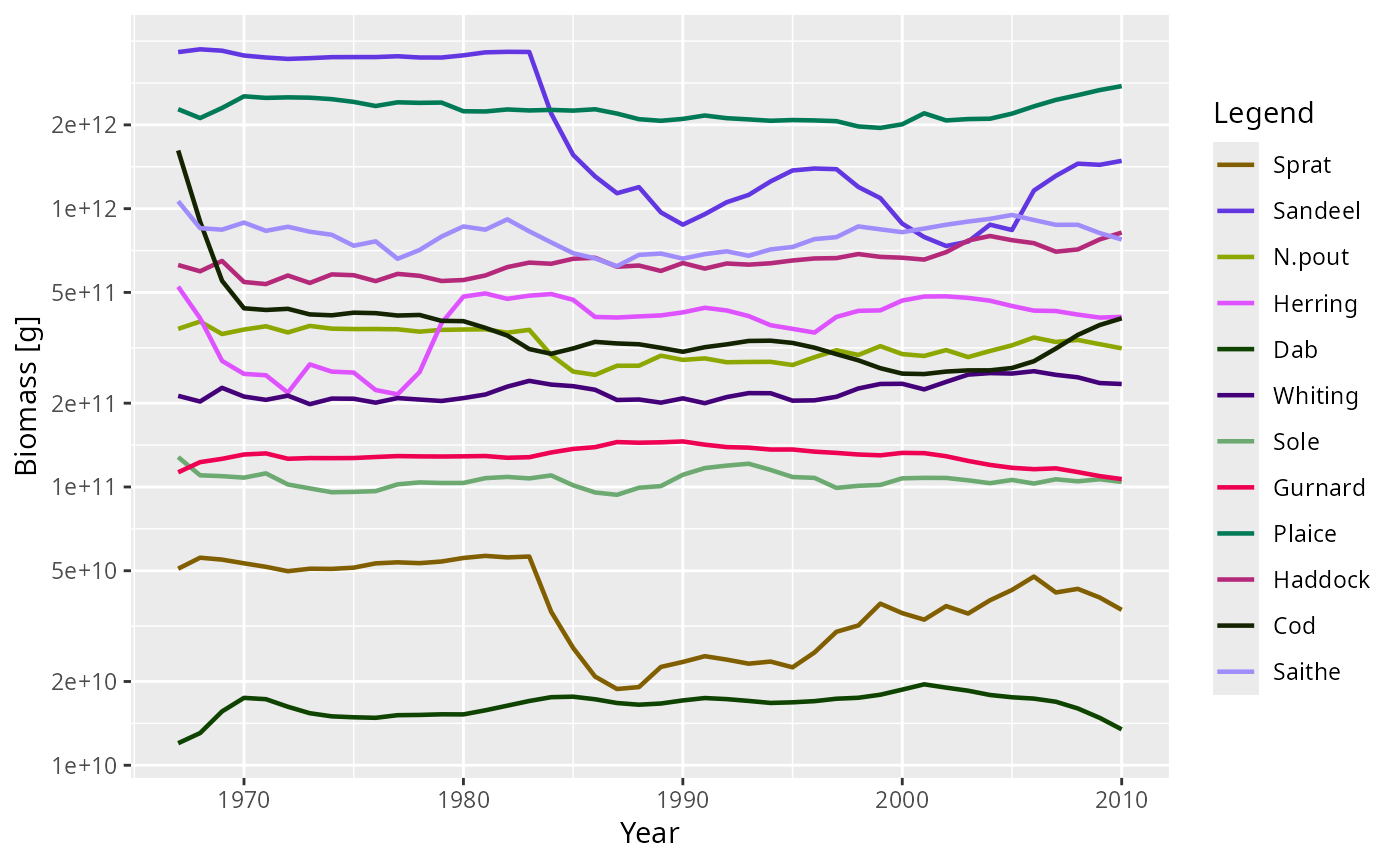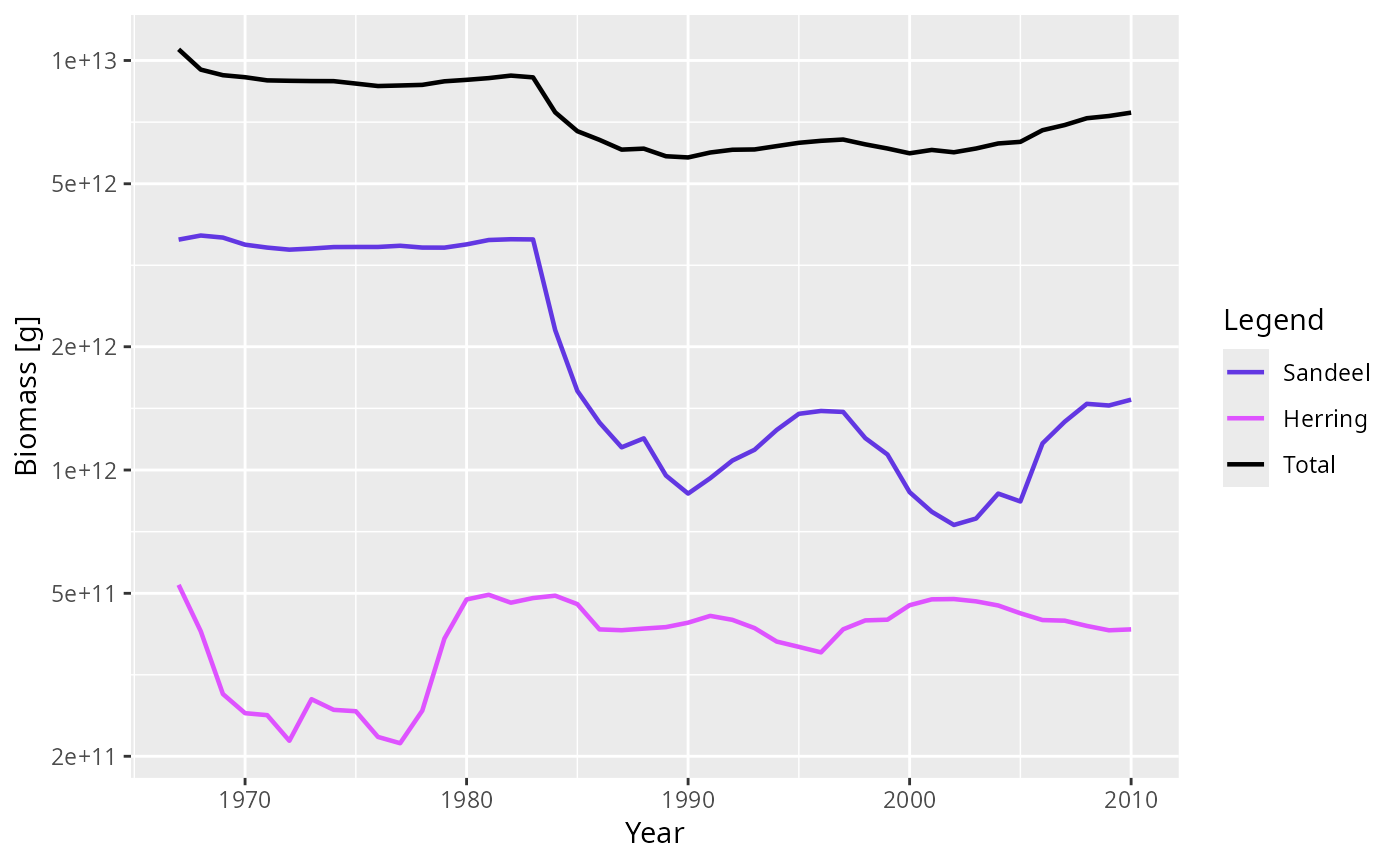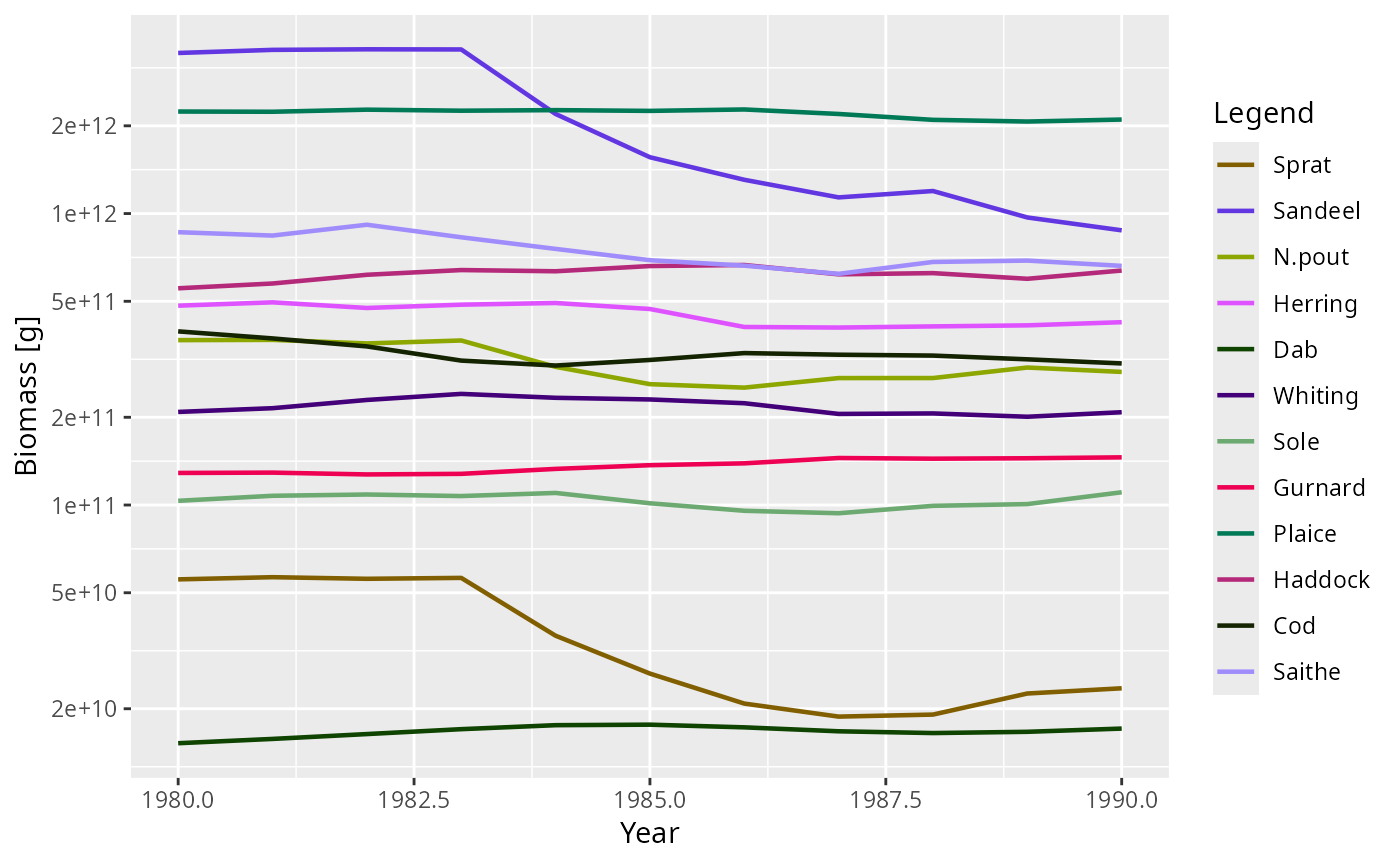After running a projection, the biomass of each species can be plotted against time. The biomass is calculated within user defined size limits (min_w, max_w, min_l, max_l, see getBiomass()).

## Usage

plotBiomass(
sim,
species = NULL,
start_time,
end_time,
y_ticks = 6,
ylim = c(NA, NA),
total = FALSE,
background = TRUE,
highlight = NULL,
return_data = FALSE,
...
)

plotlyBiomass(
sim,
species = NULL,
start_time,
end_time,
y_ticks = 6,
ylim = c(NA, NA),
total = FALSE,
background = TRUE,
highlight = NULL,
...
)

## Arguments

sim

An object of class MizerSim

species

The species to be selected. Optional. By default all target species are selected. A vector of species names, or a numeric vector with the species indices, or a logical vector indicating for each species whether it is to be selected (TRUE) or not.

start_time

The first time to be plotted. Default is the beginning of the time series.

end_time

The last time to be plotted. Default is the end of the time series.

y_ticks

The approximate number of ticks desired on the y axis

ylim

A numeric vector of length two providing lower and upper limits for the y axis. Use NA to refer to the existing minimum or maximum. Any values below 1e-20 are always cut off.

total

A boolean value that determines whether the total biomass from all species is plotted as well. Default is FALSE.

background

A boolean value that determines whether background species are included. Ignored if the model does not contain background species. Default is TRUE.

highlight

Name or vector of names of the species to be highlighted.

return_data

A boolean value that determines whether the formatted data used for the plot is returned instead of the plot itself. Default value is FALSE

...

Arguments passed on to get_size_range_array

min_w

Smallest weight in size range. Defaults to smallest weight in the model.

max_w

Largest weight in size range. Defaults to largest weight in the model.

min_l

Smallest length in size range. If supplied, this takes precedence over min_w.

max_l

Largest length in size range. If supplied, this takes precedence over max_w.

## Value

A ggplot2 object, unless return_data = TRUE, in which case a data frame with the four variables 'Year', 'Biomass', 'Species', 'Legend' is returned.

plotting_functions, getBiomass()

Other plotting functions: animateSpectra(), plot,MizerSim,missing-method, plotDiet(), plotFMort(), plotFeedingLevel(), plotGrowthCurves(), plotPredMort(), plotSpectra(), plotYieldGear(), plotYield(), plotting_functions

## Examples

# \donttest{
plotBiomass(NS_sim)plotBiomass(NS_sim, species = c("Sandeel", "Herring"), total = TRUE)plotBiomass(NS_sim, start_time = 1980, end_time = 1990)# Returning the data frame
fr <- plotBiomass(NS_sim, return_data = TRUE)
str(fr)
#> 'data.frame':	528 obs. of  4 variables:
#>  $Year : int 1967 1968 1969 1970 1971 1972 1973 1974 1975 1976 ... #>$ Biomass: num  5.08e+10 5.57e+10 5.48e+10 5.32e+10 5.16e+10 ...
#>  $Species: Factor w/ 12 levels "Sprat","Sandeel",..: 1 1 1 1 1 1 1 1 1 1 ... #>$ Legend : Factor w/ 12 levels "Sprat","Sandeel",..: 1 1 1 1 1 1 1 1 1 1 ...
# }